What are Precedence Functions in compiler design?

Compiler DesignProgramming LanguagesComputer Programming

Precedence relations between any two operators or symbols in the precedence table can be converted to two precedence functions f & g that map terminals symbols to integers.

• If a <. b, then f (a) <. g (b)
• If a = b, then f (a) =. g (b)
• If a .> b, then f (a) .> g (b)

Here a, b represents terminal symbols. f (a) and g (b) represents the precedence functions that have an integer value.

Computations of Precedence Functions

• For each terminal a, create the symbol fa&ga.
• Make a node for each symbol.

If a =. b, then fa & gb are in same group or node.

If a =. b & c =. b, then fa & fc must be in same group or node.

• (a) If a <. b, Mark an edge from gb to fa.

(b) If a .>b, Mark an edge from fa to gb.

• If the graph constructed has a cycle, then no precedence functions exist.
• If there are no cycles.

(a) fa = Length of longest path beginning at the group of fa.

(b) ga = Length of the longest path from the group of ga.

Example1 − Construct precedence graph & precedence function for the following table.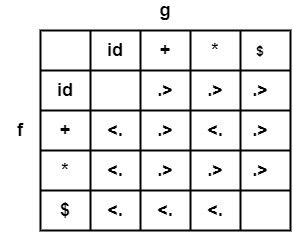Solution

Step1 − Create Symbols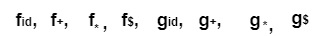Step2 − No symbol has equal precedence, as can be seen in the given table; therefore, each symbol will remain in a different node.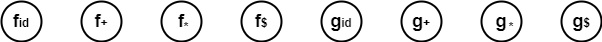Step3 − If a <. b, create an edge from fa → ga

If a .>b, create an edge from gb → fa

Since, $<. +,*, id. therefore, make an edge from g+, g*, gid to fs Similarity + <. ,∗, id. ∴ make an edge from g*, gid to f+ Similarity * <. id. Therefore, Mark an edge from gid to f*. Since, +,*, id . >$ therefore, Mark an edge from f+, f*, fid to gs.

Similarity +,*, id . > +. Mark an edge from f+, f*, fid to g+.

Similarity *, id . > *. Mark an edge from f*, fid to g.

Combining all the edges we get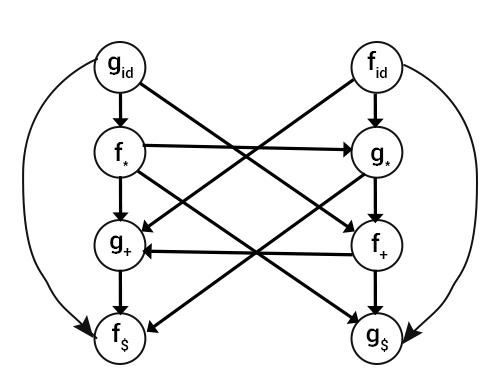Step4 − Computing the maximum length of the path from each node, we get the following precedence functions

 Id + * $F 4 2 4 0 G 5 1 3 0 Example2 − Construct precedence graph & precedence function for the following table.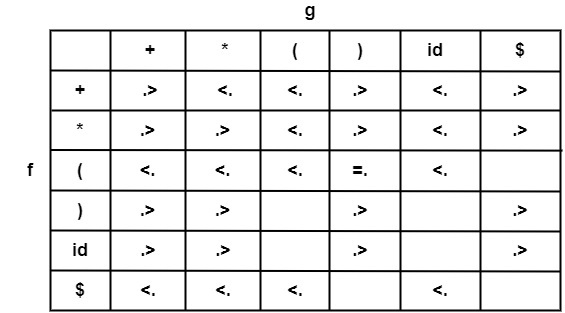Solution As we have (=.). Therefore f & g will be in the same group. Computation of precedence graph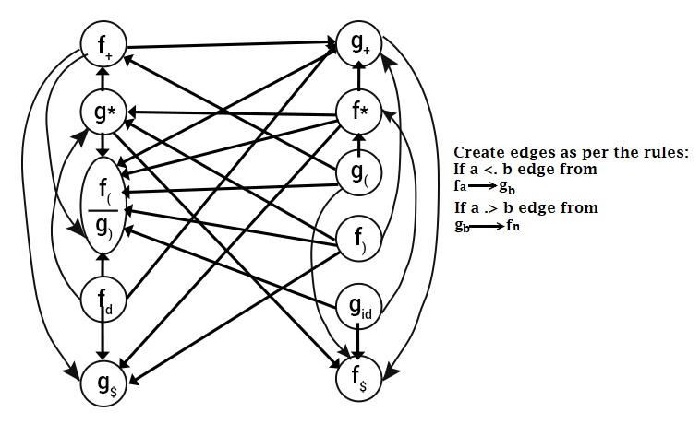Computation of Precedence Function Table Since f$ and g$have no outgoing edges, f($ ) = g($) = 0. Since f and g have no outgoing edges, f(( ) = g( )) = 0. For all others, compute the path of the longest length starting from it. For Example − Take f+, It has three outgoing edges and traces out its paths. f+ → g$

f+ → f(

f+ → g+ → f( and f+ → g+ → f$Select the path of maximum length, and the length is 2. Hence, f+ = 2. computing all paths of f and g, we get Precedence table  + * ( ) id$ F 2 4 0 4 4 0 G 1 3 5 0 5 0